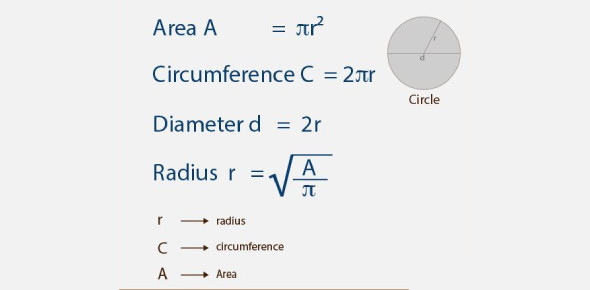Area And Circumference Quiz! Math Trivia

10 Questions | Total Attempts: 139SettingsToday in this quiz, we are going to focus on the circumference of a circle. The formula for getting the circumference of a circle is somehow similar to finding its area. Do they get you all confused, or do you know how to get the solutions when asked? The best way to test out how true your answer is will be through you taking this quiz. Give it a shot and see how much more practice you might need. All the best!

• 1.
If you wanted to find the distance around a triangle or a square, what would you be looking for?
• A.

Area

• B.

Circumference

• C.

Perimeter

• D.

Space

• 2.
A parallelogram has an area of 75 square feet. Find the base if the height is 3 feet.
• A.

25 ft

• B.

5 ft

• C.

35 ft

• D.

45.5 ft

• 3.
Find the perimeter of a square that has one side measuring 5 cm.
• A.

13 cm

• B.

10 cm

• C.

20 cm

• D.

15 cm

• 4.
Find the area of a triangle whose base measures 8 feet and whose height measures 5 feet.
• A.

20 square feet

• B.

40 square feet

• C.

13 square feet

• D.

10 square feet

• 5.
A clock has a radius of 5 inches. Which expression could be used to find the circumference of the clock?
• A.

5 times pi

• B.

10 times pi

• C.

25 times pi

• D.

5 divided by pi

• 6.
How do you find the circumference of a circle?
• A.

Base x height

• B.

• C.

Pi x diameter

• D.

• 7.
How do you find the area of a circle?
• A.

Pi x diameter

• B.

Pi x circumference

• C.

• D.

• 8.
How do you find the area of a triangle?
• A.

1/2 base x height

• B.

Base x height

• C.

Base + height

• D.

Base/height

• 9.
How do you find the area of a rectangle?
• A.

Length x width

• B.

1/2 length x width

• C.

Diameter x width

• D.

• 10.
How do you find the area of a square?
• A.

• B.

Side x 4

• C.

Side x side

• D.

Side x side x side

Related TopicsBack to top